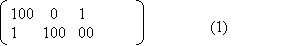PCP News
• PCPSolver version 0.0.3 is released with source code. README (Nov 16, 2003)
• PCPSolver version 0.0.2 is released with source code. (April 25, 2003)
• I published a paper named Tackling Post's Correspondence Problem in the Third International Conference on Computer and Games in 2002. (July 26, 2002)
• My Master's thesis about solving PCP is now available. (March 7, 2002)

What's Post's Correspondence Problem (PCP)?

Definition:   Given an alphabet S, one instance of Post's correspondence problem of size s is a finite set of pairs of strings (gi , hi) ( i = 1...s s>=1) over the alphabet S.  A solution of length n >= 1 to this instance is a sequence i1 i2 ... in of selections such that the strings gi1gi2 ... gin and hi1hi2 ... hin formed by concatenation are identical.

Width of a PCP instance is the length of the longest string in gi and hi (i = 1, 2, ... , s). Pair i is the short name for pair (gi , hi), where gi and hi are the top string and bottom string of the pair respectively. Mostly, people are interested in optimal solution, which has the shortest length over all possible solutions to an instance. The corresponding length is called optimal length. We use the word hard or difficult to describe instances whose optimal lengths are very large. For simplicity, we restrict the alphabet S to {0, 1}, and it is easy to transform other alphabets to their equivalent binary format.

To describe subclasses of Posts Correspondence Problem, we use PCP[s] to represent the set of all PCP instances of size s, and PCP[s, w] the set of all PCP instances of size s and width w.

For convenience, we use a matrix of 2 rows and s columns to represent instances of PCP[s], where string gi is located at (i , 1) and hi at (i , 2). The following is the matrix representation of the instance {{100, 1}, {0, 100}, {1, 00}} in PCP[3,3].Let's consider the result of selections of pair 1, 3, 1, 1, 3, 2, 2 accordingly. They can be shown in the following table with each selection assigned a different color:

 (1)1001 (3)100 (1)1001 (1)1001 (3)100 (2)0100 (2)0100

After the elimination of blanks and concatenation of strings in the top and bottom separately, it turns to:

1001100100100
1001100100100

Now, the string in the top is identical to the one in the bottom; therefore, these selections form a solution to PCP (1). When all combinations of up to 7 selections of pairs are tested, this solution is thus proven to be the unique optimal solution to this instance.

PCP's theoretical place
Post's correspondence problem was first raised by Post in 1946 as undecidable problem . It is frequently used in the literature as a basis to prove the undecidabily of other problems. The problem of size 2 has been proved decidable . Some researchers in Finland found a simpler proof . The problem of size 7 has been proved undecidable . Now the decidablility of problems with size between 3 and 6 is still pending.

 Post, E., L., A variant of a recursively unsolvable problem,Bull. of the Am. Math. Soc., 52, 1946.
 Ehrenfeucht, A., Karhumaki, J. and Rozenberg, G., The (generalized) post correspondece problem with lists consisting of two words is decidable, Theoret. Comput. Sci.,21, 2,1982.
 Vesa Halava, Tero Harju and Mika Hirvensalo, Binary (Generalized) Post Correspondence Problem, TUCS Technical Report No. 357, August 2000. [PS file]
 Y. Matiyasevich and G.Senizergues, Decision problems for semi-Thue systems with a few rules, Proceedings, 11th Anual IEEE Symposium on Logic in Computer Science, 1996. [PS file]

Introduction
What's PCP?
PCP's theoretical place

Solving hard PCP instances
Bidirectional probing
Heuristic pruning
Cache scheme
Depth-first iterative deepening
Tail recursion removal

Instances having no solutions
Filters
Exclusion method
Pattern method

Hard instances list
The known hard instances
Data of the solutions

Documents
Presentation slides
PCP Instances